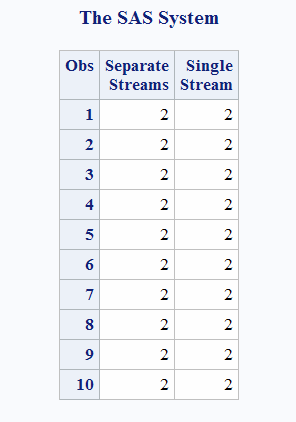# CALL RANTBL Routine

Returns a random variate from a tabled probability distribution.

 Category: Random Number

## Syntax

CALL RANTBL(seed,p1,...pi,...pn,x);

### Required Arguments

#### seed

is the seed value. A new value for seed is returned each time CALL RANTBL is executed.

 Range seed < 231 - 1 Note If seed ≤ 0, the time of day is used to initialize the seed stream. See Seed Values and Comparison of Seed Values in Random-Number Functions and CALL Routines

#### pi

is a numeric SAS value.

 Range 0 ≤ pi ≤ 1 for 0 < i ≤ n

#### x

is a numeric SAS variable. A new value for the random variate x is returned each time CALL RANTBL is executed.

## Details

The CALL RANTBL routine updates seed and returns a variate x generated from the probability mass function defined by p1 through pn.
By adjusting the seeds, you can force streams of variates to agree or disagree for some or all of the observations in the same, or in subsequent, DATA steps.
An inverse transform method applied to a RANUNI uniform variate is used. The CALL RANTBL routine returns these data:
$equation$
If, for some index j<n,
$equation$
RANTBL returns only the indices 1 through j, with the probability of occurrence of the index j equal to
$equation$
For a discussion and example of an effective use of the random number CALL routines, see Starting, Stopping, and Restarting a Stream.

## Comparisons

The CALL RANTBL routine gives greater control of the seed and random number streams than does the RANTBL function.

## Example

This example uses the CALL RANTBL routine:
```data u1(keep=x);
seed = 104;
do i = 1 to 5;
call rantbl(seed, .02, x);
output;
end;
call symputx('seed', seed);
run;
data u2(keep=x);
seed = &seed;
do i = 1 to 5;
call rantbl(seed, .02, x);
output;
end;
run;
data all;
set u1 u2;
z = rantbl(104, .02);
run;
proc print label;
label x = 'Separate Streams' z = 'Single Stream';
run;```
Output from the CALL RANTBL Routine